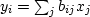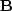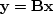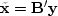# FAMILIAR OPERATORS

The operationis multiplication of a matrixtimes a vector.The adjoint operation is.The operation adjoint to multiplying by a matrix is multiplying by the transposed matrix (unless the matrix has complex elements, in which case we need the complex-conjugated transpose). The following pseudocode does matrix multiplicationand multiplication by the transpose matrix:


if operator itself
then erase y
then erase x
do iy = 1, ny {
do ix = 1, nx {
if operator itself
y(iy) = y(iy) + b(iy,ix)x(ix)
x(ix) = x(ix) + b(iy,ix)y(iy)
}}


Notice that the bottom line'' in the program is that x and y are simply interchanged. The above example is a prototype of many to follow, so observe carefully the similarities and differences between the operation and its adjoint.

A formal program for matrix multiply and its adjoint is found below. The first step is erasing the output. That may seem like too trivial a function to put in a separate library routine, but, at last count, 15 other routines in this book use the output-erasing subroutine adjnull() below.

subroutine adjnull( adj, add, x, nx,  y, ny )
real                          x( nx), y( ny )
do iy= 1, ny
y(iy) = 0.
else
do ix= 1, nx
x(ix) = 0.
return; end


The subroutine matmult()for matrix multiply and its adjoint exhibits a style that we will use repeatedly.

# matrix multiply and its adjoint
#
subroutine matmult( adj, bb,        nx,x,  ny,y)
integer ix, iy,    adj,            nx,    ny
real                    bb(ny,nx), x(nx), y(ny)
do ix= 1, nx {
do iy= 1, ny {
y(iy) = y(iy) + bb(iy,ix) * x(ix)
else
x(ix) = x(ix) + bb(iy,ix) * y(iy)
}}
return; end